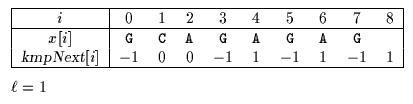Next: Not So Naive algorithm Up: ESMAJ Prev: Galil-Giancarlo algorithm

# Apostolico-Crochemore algorithm

Main features
• preprocessing phase in O(m) time and space complexity;
• searching phase in O(n) time complexity;
• performsn text character comparisons in the worst case.
Description

The Apostolico-Crochemore uses the kmpNext shift table (see chapter Knuth, Morris and Pratt algorithm) to compute the shifts.

Let=0 if x is a power of a single character (x=cm with c in) andbe equal to the position of the first character of x different from x otherwise (x=abu for a, b in, u in* and ab). During each attempt the comparisons are made with pattern positions in the following order:,+1, ... , m-2, m-1, 0, 1, ... ,-1.

During the searching phase we consider triple of the form (i, j, k) where:the window is positioned on the text factor y[j .. j+m-1];0kand x[0 .. k-1]=y[j .. j+k-1];i < m and x[.. i-1]=y[j+.. i+j-1].

The initial triple is (,0,0).Figure 11.1: At each attempt of the Apostolico-Crochemore algorithm we consider a triple (i,j,k).

We now explain how to compute the next triple after (i,j,k) has been computed.

Three cases arise depending on the value of i:i =If x[i] = y[i+j] then the next triple is (i+1, j, k). If x[i]y[i+j] then the next triple is (, j+1, max{0, k-1}).< i < m If x[i] = y[i+j] then the next triple is (i+1, j, k). If x[i]y[i+j] then two cases arise depending on the value of kmpNext[i]: kmpNext[i]: then the next triple is (, i+j-kmpNext[i], max{0, kmpNext[i]}) kmpNext[i] >: then the next triple is (kmpNext[i], i+j-kmpNext[i],)i =m If k <and x[k]=y[j+k] then the next triple is (i, j, k+1). Otherwise either k <and x[k]y[j+k], or k=. If k=an occurrence of x is reported. In both cases the next triple is computed in the same manner as in the case where< i < m.

The preprocessing phase consists in computing the table kmpNext and the integer. It can be done in O(m) space and time. The searching phase is in O(n) time complexity and furthermore the Apostolico-Crochemore algorithm performs at mostn text character comparisons in the worst case.

The C code

The function preKmp is given chapter Knuth, Morris and Pratt algorithm.

```void AXAMAC(char *x, int m, char *y, int n) {
int i, j, k, ell, kmpNext[XSIZE];

/* Preprocessing */
preKmp(x, m, kmpNext);
for (ell = 1; x[ell - 1] == x[ell]; ell++);
if (ell == m)
ell = 0;

/* Searching */
i = ell;
j = k = 0;
while (j <= n - m) {
while (i < m && x[i] == y[i + j])
++i;
if (i >= m) {
while (k < ell && x[k] == y[j + k])
++k;
if (k >= ell)
OUTPUT(j);
}
j += (i - kmpNext[i]);
if (i == ell)
k = MAX(0, k - 1);
else
if (kmpNext[i] <= ell) {
k = MAX(0, kmpNext[i]);
i = ell;
}
else {
k = ell;
i = kmpNext[i];
}
}
}

```
The example

Preprocessing phasekmpNext table used by Apostolico-Crochemore algorithm

Searching phase

References
• APOSTOLICO A., CROCHEMORE M., 1991, Optimal canonization of all substrings of a string, Information and Computation 95(1):76-95.
• HANCART, C., 1993. Analyse exacte et en moyenne d'algorithmes de recherche d'un motif dans un texte, Ph. D. Thesis, University Paris 7, France.Next: Not So Naive algorithm Up: ESMAJ Prev: Galil-Giancarlo algorithm

e-mails: {Christian.Charras, Thierry.Lecroq }@laposte.net
Tue Jan 14 15:03:31 MET 1997